# Boolean-valued model

(diff) ← Older revision | Latest revision (diff) | Newer revision → (diff)

A model defined as follows. Letbe the signature of some first-order language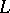with one kind of variables, i.e.is the set of symbols of functions and predicates. A Boolean-valued model then is a triple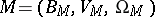, where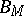is a non-degenerate Boolean algebra,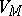is a non-empty set, andis a function defined onsuch that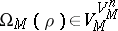ifis an-place function symbol, andifis an-place predicate symbol. The symboldenotes the set of all functions defined on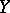with values inand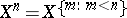, where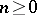is a natural number. The Boolean algebrais called the set of truth values of the model. The setis called the universe of. A Boolean-valued modelis also called a-model if the set of truth values is the Boolean algebra,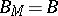. If a Boolean algebrais a two-element algebra (i.e.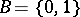), then the-modelis the classical two-valued model.

Let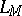be a language, complemented by new individual constants: each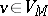having its own individual constant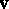. Letbe a-model and let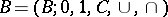be a complete Boolean algebra; the equalities 1)–8) below then define the value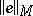of each closed expression(i.e. of a formula or a term without free variables) of:

1)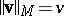, where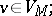2)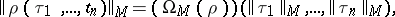whereare closed terms andis an-place function or predicate symbol;

3)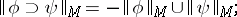4)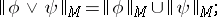5)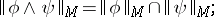6)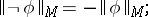7)8)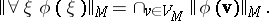The relations 1)–8) define the valuefor certain non-complete Boolean algebras as well; the only condition is that the infinite unions and intersections in 7) and 8) exist. The concept of a Boolean-valued model can also be introduced for languages with more than one kind of variables. In such a case each kind of variable has its own domain of variation.

A closed formulais said to be true in a-model(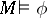) if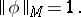A-modelis said to be a model of a theoryiffor all axiomsof. If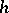is a homomorphism of a Boolean algebrainto a Boolean algebra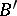preserving infinite unions and intersections, then there exists amodelsuch thatfor each closed formulaof. If the universe of a modelis countable, then there exists a homomorphisminto the Boolean algebra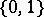, under whichis transformed into the classical two-valued modelsuch that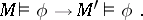It has been shown that a theoryis consistent if and only if it has a Boolean-valued model. This theorem forms the basis of the application of the theory of Boolean-valued models to problems of the consistency of axiomatic theories.

If the Boolean-valued model of a theoryis constructed by means of another axiomatic theory, then one obtains the statement on the consistency ofrelative to. Thus, the result due to P. Cohen on the consistency of the theory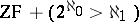relative to ZF is obtained by constructing the respective Boolean-valued model by means of the system ZF (cf. Forcing method). The construction of the Cohen forcing relationis equivalent to that of a Boolean-valued modelsuch thatHow to Cite This Entry:
Boolean-valued model. Encyclopedia of Mathematics. URL: http://encyclopediaofmath.org/index.php?title=Boolean-valued_model&oldid=17991
This article was adapted from an original article by V.N. Grishin (originator), which appeared in Encyclopedia of Mathematics - ISBN 1402006098. See original article